In the figure above, ABCD is a quadrilateral ...

### Related Test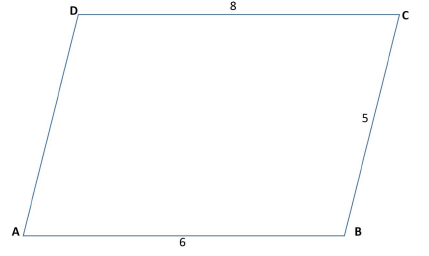In the figure above, ABCD is a quadrilateral in which sides AB and CD are parallel. What is the area of the quadrilateral?
(1) The distance between sides AB and CD is 4 units
(2) The length of side AD is √17 units
• a)
Statement (1) ALONE is sufficient, but statement (2) alone is
• b)
Statement (2) ALONE is sufficient, but statement (1) alone is
• c)
BOTH statements (1) and (2) TOGETHER are sufficient to
• d)
EACH statement ALONE is sufficient to answer the question
• e)
Statements (1) and (2) TOGETHER are NOT sufficient to
problem are needed.Pioneer AcademyStep 1 & 2: Understand Question and Draw Inference
Given:
• In quadrilateral ABCD, AB || CD
• ABCD is a trapezium
To find: Area of quadrilateral ABCD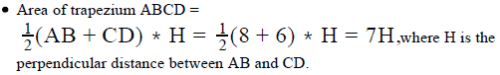So, to find the area of trapezium, we need to find the value of H
Step 3 : Analyze Statement 1 independent
• Statement 1 is:
• H = 4
• This gives the value of H. Hence, Statment 1 is sufficient to find the area.
Step 4 : Analyze Statement 2 independent
• Statement 2 is:
• Let’s drop perpendiculars DE and CF on side AB.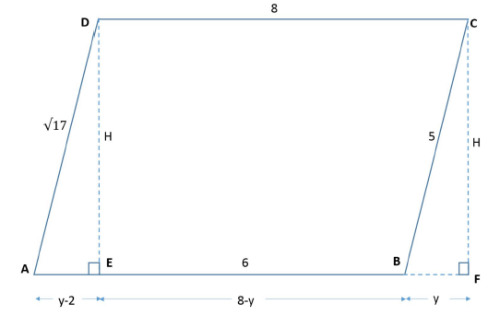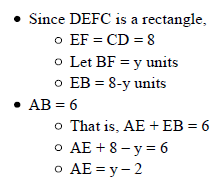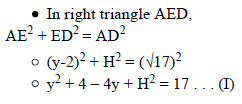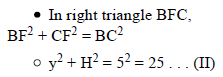• Put (II) in (I):
25 + 4 – 4y = 17
• 4y = 12
• y = 3 . . . (III)

• Put (III) in (II)
32 + H2 = 25
• H2 = 16
• H = 4 (rejecting negative value since length cannot be negative)

• Since we now have a unique value of H, we can find a uniqu e value of the area as well.
• Hence Statement 2 is also sufficient to answer the question
Step 5: Analyze Both Statements Together (if needed)
Since we’ve already arrived at a unique answer in each of Steps 3 and 4, this
step is not required
Therefore, the correct answer is Option D.

 1 Crore+ students have signed up on EduRev. Have you?Mittul Madaan
Step 1 & 2: Understand Question and Draw Inference
Given:
• In quadrilateral ABCD, AB || CD
• ABCD is a trapezium
To find: Area of quadrilateral ABCDSo, to find the area of trapezium, we need to find the value of H
Step 3 : Analyze Statement 1 independent
• Statement 1 is:
• H = 4
• This gives the value of H. Hence, Statment 1 is sufficient to find the area.
Step 4 : Analyze Statement 2 independent
• Statement 2 is:
• Let’s drop perpendiculars DE and CF on side AB.• Put (II) in (I):
25 + 4 – 4y = 17
• 4y = 12
• y = 3 . . . (III)

• Put (III) in (II)
32 + H2 = 25
• H2 = 16
• H = 4 (rejecting negative value since length cannot be negative)

• Since we now have a unique value of H, we can find a uniqu e value of the area as well.
• Hence Statement 2 is also sufficient to answer the question
Step 5: Analyze Both Statements Together (if needed)
Since we’ve already arrived at a unique answer in each of Steps 3 and 4, this
step is not required
Therefore, the correct answer is Option D.View courses related to this question Explore GMAT courses
 Explore GMAT coursesView courses related to this question• ### Directions: Each GMAT Data Sufficiency problem consists of a question and t... more(Scan QR code)